# 成分句法分析

Posted by lili on

## 上下文无关文法(Context-Free Grammars/PCFGs)

• N是一个有限的非终结符集合
• $\Sigma$是一个有限的终结符集合(字母表)
• R是一个有限的产生式规则集合，每一个产生式规则的形式为$X \rightarrow Y_1Y_2…Y_n$，其中$X \in N$，$Y_i \in N \cup \Sigma$。
• $S \in N$是一个特殊的开始符号

$\begin{split} S \rightarrow \text{NP VP} \\ \text{NN} \rightarrow man \end{split}$

$\begin{split} \text{NN} \rightarrow man \\ S \rightarrow \text{NP} \end{split}$

$\begin{split} \text{VP} \rightarrow \text{John Vt Mary} \\ \text{NP} \rightarrow \text{the NN} \end{split}$

$\begin{split} \text{VP} \rightarrow \epsilon \\ \text{VP} \rightarrow \epsilon \end{split}$

• $s_1=S$，也就是说$s_1$是只包含开始符号的字符串
• $s_n \in \Sigma^*$，即最后一个字符串只包含终结符号
• $s_i$是这么从$s_{i-1}$推导出来的——把$s_i$的右边的第一个非终结符号用一个产生式规则的右边替换得到，要求这个产生式规则的左边就是$s_i$的第一个非终结符号

$\begin{split} & s_1=S \\ & s_2= \text{NP VP} (S \rightarrow \text{NP VP}) \\ & s_3=\text{DT NN VP} (\text{NP} \rightarrow \text{DT NN})\\ & s_4=\text{the NN VP}(\text{DT} \rightarrow the) \\ & s_5=\text{the man VP}(\text{NN} \rightarrow man) \\ & s_6=\text{the man Vi}(\text{VP} \rightarrow \text{Vi}) \\ & s_7=\text{the man sleeps}(\text{Vi} \rightarrow sleeps) \end{split}$

## PCFGs的定义

$\mathcal{T}_G(s)=\{t: t \in \mathcal{T}_G, yield(t)=s \}$

$\begin{split} p(t) \ge 0 \\ \sum_{t \in \mathcal{T}_G}p(t)=1 \end{split}$

$\underset{t \in \mathcal{T}_G(s)}{argmax}p(t)$

• 怎么定义概率p(t)
• 怎么根据数据学习出概率p(t)的参数
• 根据句子s，怎么快速的计算$\underset{t \in \mathcal{T}_G(s)}{argmax}p(t)$

• 一个CFG $G=(N, \Sigma, S, R)$
• 对于R中的每一个产生式规则$\alpha \rightarrow \beta$，有对应的$q(\alpha \rightarrow \beta)$。它可以认为是在进行最左推导是把$\alpha$替换成$\beta$的概率。

$\begin{split} \underset{\alpha \rightarrow \beta \in R: \alpha=X}{\Sigma}q(\alpha \rightarrow \beta)=1 \\ q(\alpha \rightarrow \beta) \ge 0 \end{split}$

$p(t)=\prod_{i=1}^{n}q(\alpha_i \rightarrow \beta_i),\text{ 其中}\alpha_i \rightarrow \beta_i \text{是最左推导第i步用到的产生式规则}$

$\begin{split} \underset{\alpha \rightarrow \beta \in R: \alpha=VP}{\Sigma}q(\alpha \rightarrow \beta) & = q(\text{VP} \rightarrow \text{Vi}) + q(\text{VP} \rightarrow \text{Vt NP}) + q(\text{VP} \rightarrow \text{VP PP}) \\ & = 0.3 + 0.5 + 0.2 \\ & = 1.0 \end{split}$

$\begin{split} p(t) = & q(S \rightarrow \text{NP VP}) \times q(\text{NP} \rightarrow \text{DT NN}) \\ & \times q(\text{DT} \rightarrow the) \times q(\text{NN} \rightarrow dog) \times q(\text{VP} \rightarrow \text{Vi}) \times q(\text{Vi} \rightarrow sleeps) \end{split}$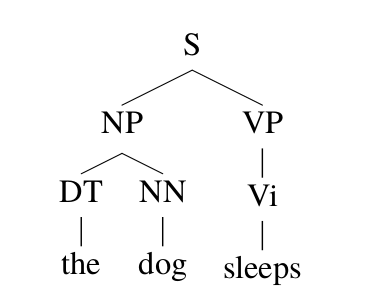图：一个最左推导对应的parse tree

PCFGs是一个产生式模型，它可以如下的产生一个句子：

• 当i=1的时候，$s_1=S$
• 如果$s_i$全是终结符号，那么过程结束，并且得到一个句子，否则：
• 找到$s_i$最左边的非终结符号，假设是X
• 根据概率分布$q(X \rightarrow \beta)$选择产生式规则$X \rightarrow \beta$
• 替换$s_i$的X为$\beta$得到$s_{i+1}$
• $i=i+1$

## PCFGs的参数估计

• N是$t_1,…,t_m$里出现过的非终结符号的集合
• $\Sigma$是$t_1,…,t_m$中出现过的终结符号的集合
• S是开始符号
• R是所有在$t_1,…,t_m$中出现过的产生式规则的集合
• q使用最大似然估计得到：

$q_{ML}(\alpha \rightarrow \beta)=\frac{Count(\alpha \rightarrow \beta)}{Count(\alpha)}$

上式中$Count(\alpha \rightarrow \beta)$是$t_1,…,t_m$里这个规则使用的次数，而$Count(\alpha)$是非终极符号$\alpha$出现的次数。

## PCFGs的Parsing

$\underset{t \in \mathcal{T}_G(s)}{argmax}p(t)$

### 乔姆斯基范式(Chomsky Normal Form/CNF)

$\begin{split} X \rightarrow Y_1Y_2, \text{ 其中}X,Y_1,Y_2 \in N \\ X \rightarrow a, \text{其中} X \in N a \in \Sigma \end{split}$

### CKY算法

$\underset{t \in \mathcal{T}_G(s)}{argmax}p(t)$

• 给定句子$x_1…x_n$，$X \in N$，$1 \le i \le j \le n$，我们定义$\mathcal{T}(i,j,X)$为所有通过X推导出$x_i…x_j$ parse tree的集合

• $\pi(i,j,X)=\underset{t \in \mathcal{T}(i,j,X)}{max}p(t)$，如果$\mathcal{T}(i,j,X)$是空集，我们定义$\pi(i,j,X)=0$

$\underset{t \in \mathcal{T}_G(s)}{max}p(t)=\pi(1,n,S)$

$\pi(i,i,X)=\begin{cases} q(X \rightarrow x_i) \text{ if }X \rightarrow x_i \in R \\ 0 \text{ 否则} \end{cases}$

$\pi(i,j,X)=\underset{\underset{s \in \{i,...,j-1\} }{X \rightarrow \text{YZ} \in R,}}{max}(q(X \rightarrow \text{YZ}) \times \pi(i,s,Y) \times \pi(s+1,j,Z))$

## 词汇化的(Lexicalized)PCFGs

### PCFGs的问题

PCFGs有两个问题：模型忽略了词汇的(lexcial)信息；忽略句法结构信息。词汇化的PCFGs用于解决第一个问题，而第二个问题我们尝试通过扩展词汇化的PCFGs来解决。比如下图的parsing tree，它的概率为：

$q(S \rightarrow \text{NP VP}) \times q(\text{VP} \rightarrow \text{VB NP}) \times q(\text{NP} \rightarrow \text{NNP}) \times q(\text{NP} \rightarrow \text{NNP}) \\ \times q(\text{NNP} \rightarrow IBM ) \times q(\text{VB} \rightarrow bought) \times q(NNP \rightarrow Lotus)$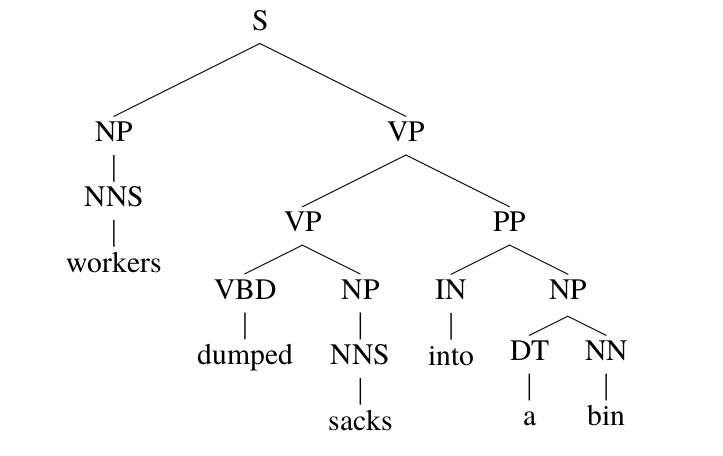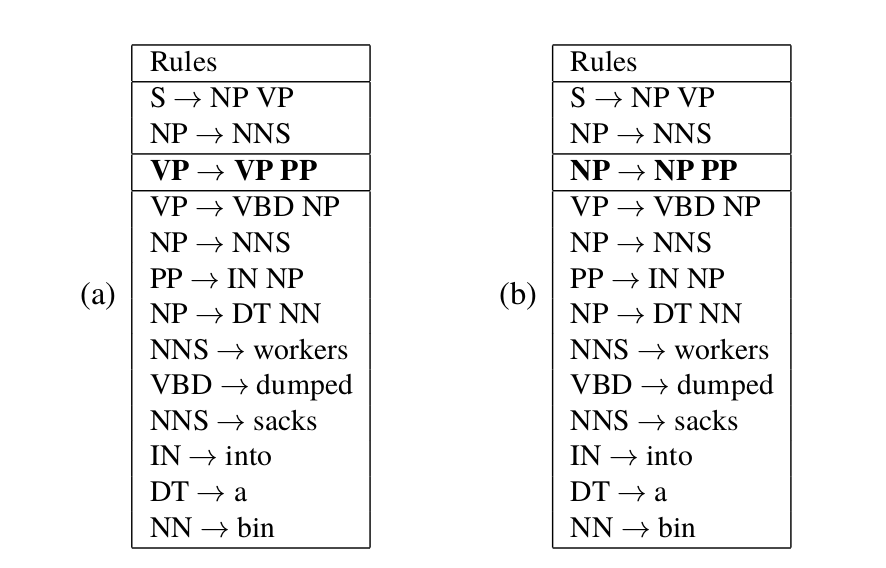图：两种parsing使用的产生式规则序列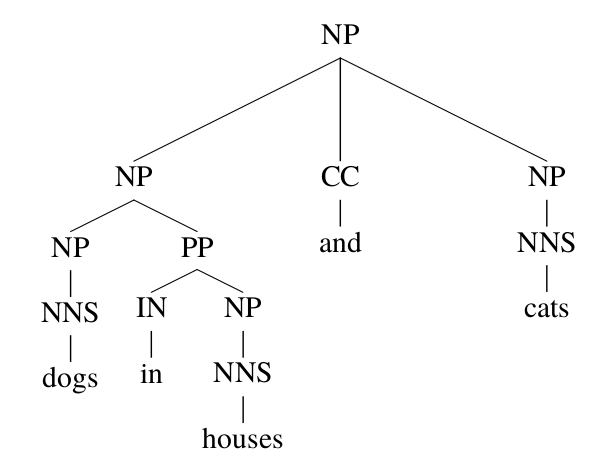PCFGs除了忽略词汇的信息之外，它也忽略树的结构信息，这类信息对于人来parsing也是很有用的。如下图所示，句子”president of a company in Africa”有两种parsing方法，这里显然是第一种更加合理——非洲某个公司的总裁。从词汇的角度，in Africa更时候修饰company和不是president。但是即使不看词汇信息，利用句子结构信息，我们也可以判断第一种的可能性更大。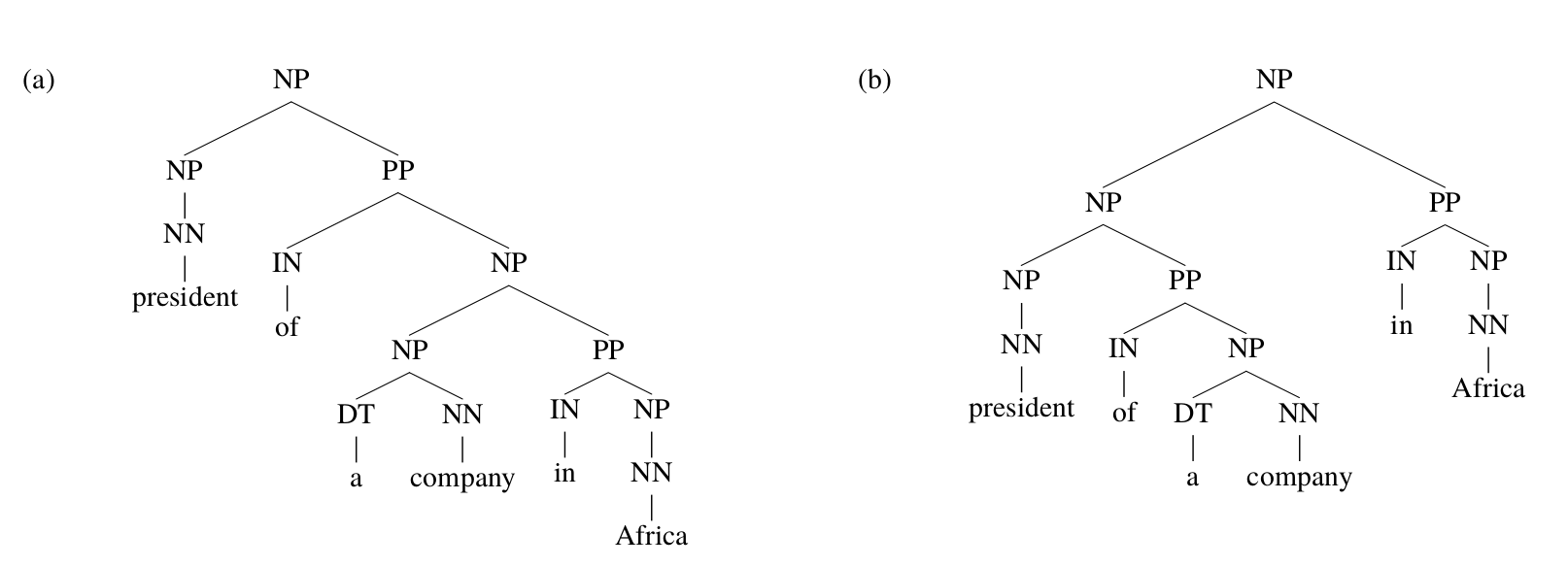图：”president of a company in Africa”的两种parsing方法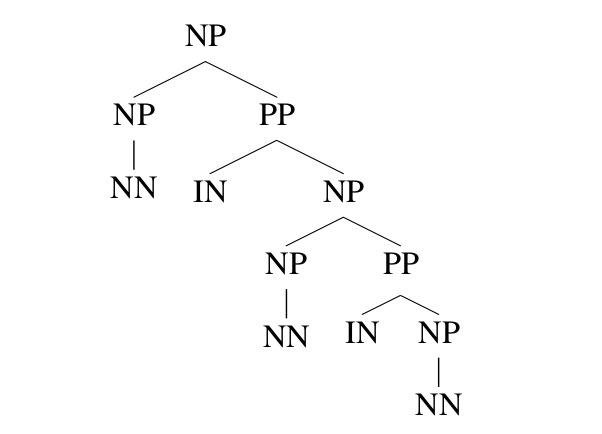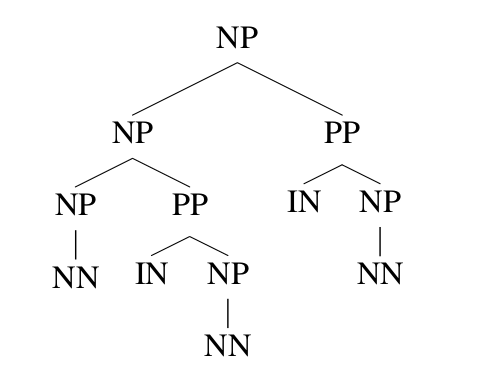### TreeBank的词汇化(Lexicalization)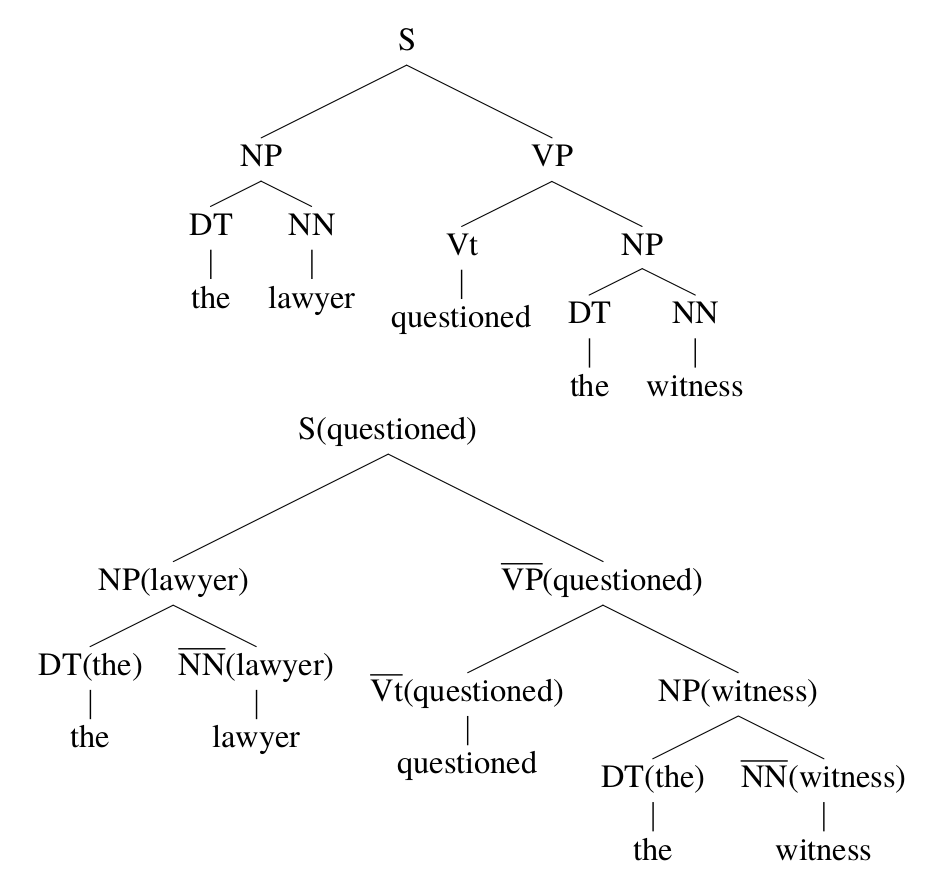图：Parsing Tree的词汇化

$S \rightarrow \text{NP VP}$

$S(questioned) \rightarrow \text{NP(lawyer) VP(questioned)}$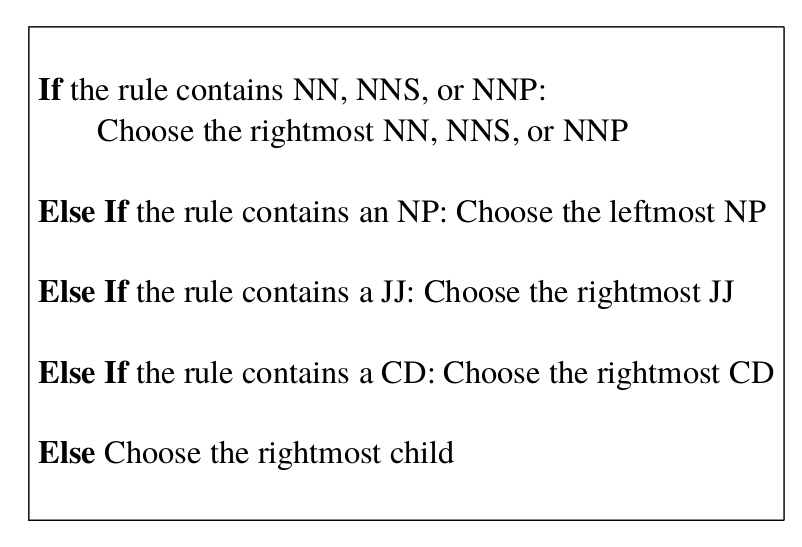图：确定产生式规则的head的一些规则示例

### 词汇化PCFGs的定义

$S \rightarrow \text{NP VP}$

$S(examined) \rightarrow \text{NP(lawyer) VP(examined)}$

• N是一个有限的非终结符号的集合
• $\Sigma$是一个有限的终结符号集合
• R是一个有限的产生式规则集合，规则只能是如下3种形式之一

$\begin{split} X(h) \rightarrow_1 Y_1 (h) Y_2 (m) \text{，其中 } X, Y_1 , Y_2 \in N , h, m \in \Sigma \\ X(h) \rightarrow_2 Y_1 (m) Y_2 (h) \text{，其中 } X, Y_1 , Y_2 \in N , h, m \in \Sigma \\ X(h) \rightarrow h \text{，其中 } X \in N , h \in \Sigma \end{split}$
• 对于任何的$r \in R$，有$q(r) \ge 0$，并且对于任何的$X \in N, h \in \Sigma$，有：

$\underset{r \in R:\text{LHS}(R)=X(h)}{\Sigma}q(r)=1$

上式中$\text{LHS}(R)$表示产生规则的左边部分，也就是$\rightarrow$左边的非终结符号X(h)

• 对于任何的$X \in N, h \in \Sigma$，有一个对应的$\gamma(X, h) \ge 0$，并且满足：

$\underset{X \in N, h \in \Sigma}{\Sigma}\gamma(X,h)=1$

$\gamma(LHS(r_1))\prod_{i=1}^{N}q(r_i)$

$\begin{split} & \text{S(questioned)} \rightarrow_2 \text{NP(lawyer) VP(questioned)} \\ & \text{NP(lawyer)} \rightarrow_2 \text{DT(the) NN(lawyer)} \\ & \text{DT(the)} \rightarrow the \\ & \text{NN(lawyer)} \rightarrow lawyer \\ & \text{VP(questioned)} \rightarrow_1 \text{Vt(questioned) NP(witness)} \\ & \text{NP(witness)} \rightarrow_2 \text{DT(the) NN(witness)} \\ & \text{DT(the)} \rightarrow the \\ & \text{NN(witness)} \rightarrow witness \\ \end{split}$

$\begin{split} & \gamma(S, questioned) \\ & q(\text{S(questioned)} \rightarrow_2 \text{NP(lawyer) VP(questioned)}) \\ & q(\text{NP(lawyer)} \rightarrow_2 \text{DT(the) NN(lawyer)}) \\ & q(\text{DT(the)} \rightarrow the) \\ & q(\text{NN(lawyer)} \rightarrow lawyer) \\ & q(\text{VP(questioned)} \rightarrow_1 \text{Vt(questioned) NP(witness)}) \\ & q(\text{NP(witness)} \rightarrow_2 \text{DT(the) NN(witness)}) \\ & q(\text{DT(the)} \rightarrow the) \\ & q(\text{NN(witness)} \rightarrow witness) \\ \end{split}$

### 词汇化PCFGs的参数估计

$\begin{split} & X=S \\ & H=examined \\ & M=lawyer \\ & R=S \rightarrow_2 \text{NP VP} \end{split}$

$\begin{split} & P (R=S \rightarrow_2 \text{NP VP}, M = lawyer|X = S, H = examined) \\ = & P (R=S \rightarrow_2 \text{NP VP}|X = S, H = examined) \\ & \times P (M = lawyer|R=S \rightarrow_2 \text{NP VP}, X = S, H = examined) \end{split}$

$\begin{split} q_{ML} (S \rightarrow_2 \text{NP VP}|S, examined)=\frac{count(R = S \rightarrow_2 \text{NP VP}, X = S, H = examined)}{count(X = S, H = examined)} \end{split}$

$P (R=S \rightarrow_2 \text{NP VP}|X = S, H = examined)=q_{ML} (S \rightarrow_2 \text{NP VP}|S, examined)$

$q_{ML}(S \rightarrow_2 \text{NP VP}|S)=\frac{count(R = S \rightarrow_2 \text{NP VP}, X = S)}{count(X = S)}$

$\begin{split} & P (R=S \rightarrow_2 \text{NP VP}|X = S, H = examined)\\ & = \lambda_1 \times q_{ML} (S \rightarrow_2 \text{NP VP}|S, examined) +(1-\lambda_1) \times q_{ML}(S \rightarrow_2 \text{NP VP}|S) \end{split}$

$\begin{split} q_{ML}(lawyer|S \rightarrow_2 \text{NP VP}, examined) & = \frac{count(M = lawyer, R = \rightarrow_2 \text{NP VP}, H = examined)}{count(R = \rightarrow_2 \text{NP VP}, H = examined)} \\ q_{ML} (lawyer|S \rightarrow_2 \text{NP VP}) & = \frac{count(M = lawyer, R = \rightarrow_2 \text{NP VP})}{count(R = S \rightarrow_2 \text{NP VP})} \end{split}$

$\begin{split} & P (M = lawyer|R \rightarrow_2 \text{NP VP}, X = S, H = examined) \\ & = \lambda_2 \times q_{ML}(lawyer|S \rightarrow_2 \text{NP VP}, examined) + (1-\lambda_2) \times q_{ML} (lawyer|S \rightarrow_2 \text{NP VP}) \end{split}$

$\begin{split} & q(S(examined) \rightarrow_2 \text{NP(lawyer) VP(examined)}) \\ = & (\lambda_1 \times q_{ML} (S \rightarrow_2 \text{NP VP}|S, examined) + (1 − \lambda_1 ) \times q_{ML}(S \rightarrow_2 \text{NP VP}|S)) \\ & \times (\lambda_2 × q_{ML}(lawyer|S \rightarrow_2 \text{NP VP}, examined) + (1 − \lambda_2 ) \times q_{ML} (lawyer|S \rightarrow_2 \text{NP VP})) \end{split}$

### 词汇化PCFGs的Parsing算法

$\pi(i,i,i,X)=q(X(x_i) \rightarrow x_i)$

$\pi(1, 1, 1, \text{NNS}) = q(\text{NNS(workers)} \rightarrow workers)$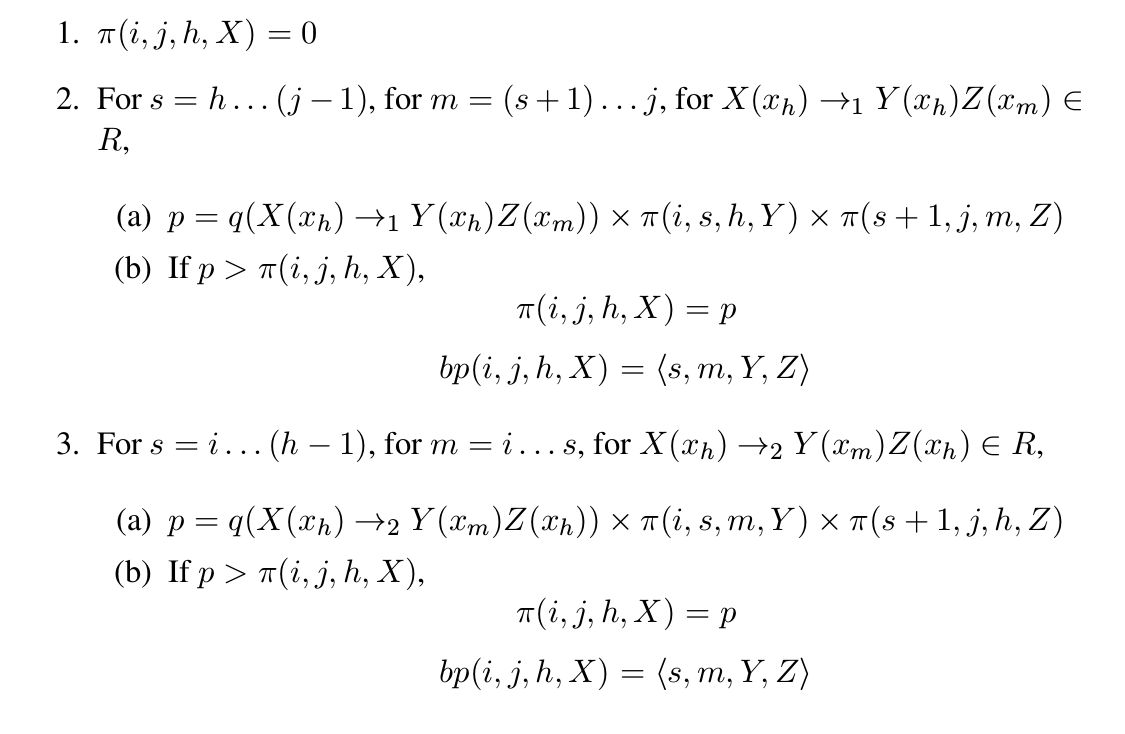图：$\pi(i,j,k,X)$的计算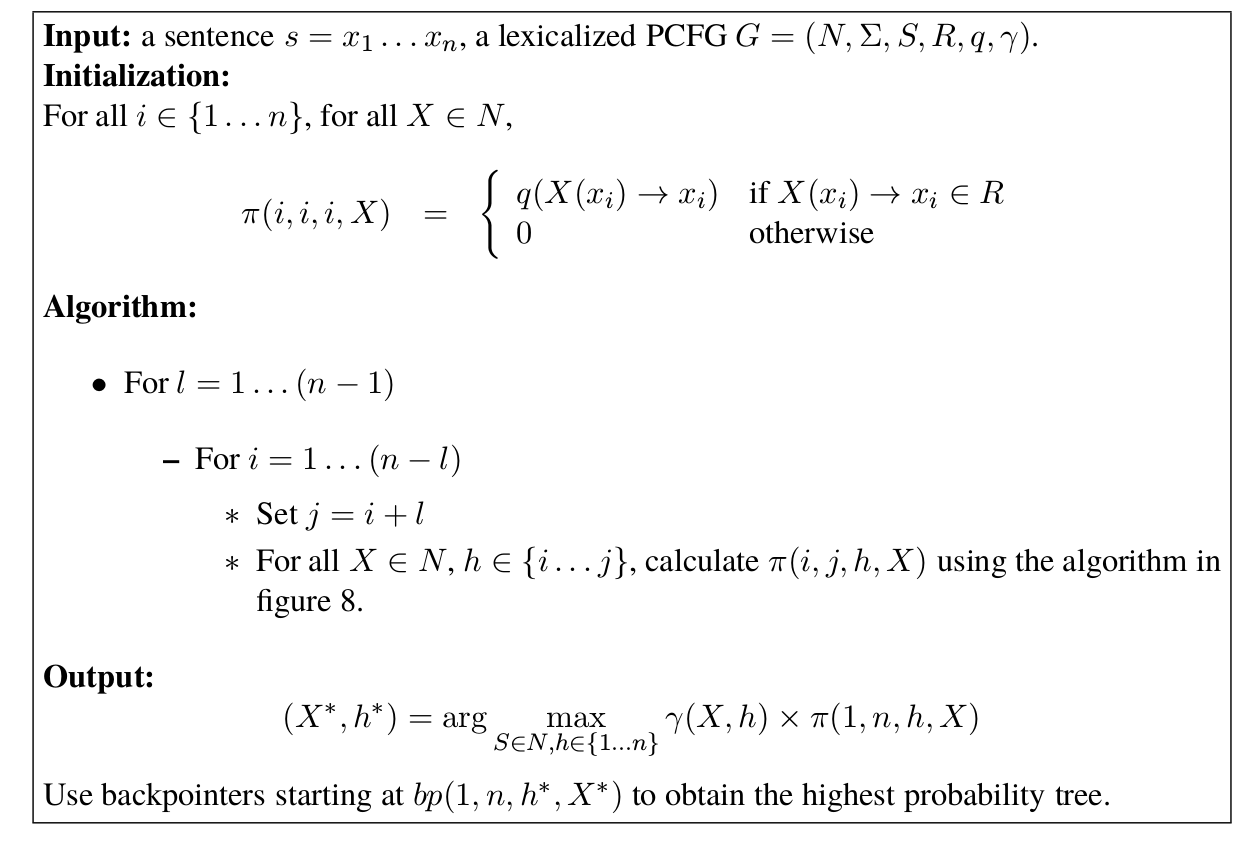图：词汇化PCFGs的CKY算法

## 成分句法分析的效果评估

$F_1=\frac{1}{\frac{1}{precision}+\frac{1}{recall}}=\frac{2 \cdot precision \cdot recall}{precision+recall}$

候选(candidate)  正确(gold)
X:a      X:a
Y:b      Z:b
Z:cd     V:cd
--       Y:bcd
W:abcd   W:abcd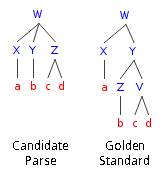图：模型的parse树和正确(参考)的parse树